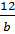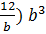### Sample Problem

Which of the following equations passes through the points (1,12) and (3,108)?

#### Solution

y = a×bx

If x=1 y=12,  12 = ab

a =;

If x=3 y=108, 108 = ab3

108 = (108 = 12b2

9 = b2

3 = b

a=4

So  y = 4×3x

The equations (B) is the correct answer.